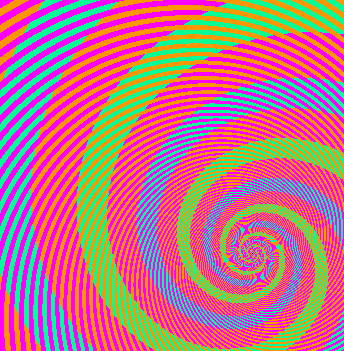# Optical illusion, mathematical illusion

Someone sent me a link to an optical illusion while I was working on a math problem. The two things turned out to be related.

In the image below, what look like blues spiral and green spirals are actually exactly the same color. The spiral that looks blue is actually green inside, but the magenta stripes across it make the green look blue. I know you don’t believe me; I didn’t believe it either. You can open it in an image editor and use a color selector to examine it for yourself.My math problem was also a case of two things that look different even though they are not. Maybe you can think back to a time as a student when you knew your answer was correct even though it didn’t match the answer in the back of the book. The two answers were equivalent but written differently. In an algebra class you might answer 5 / √ 3 when the book has 5 √ 3 / 3. In trig class you might answer 1 – cos2x when the book has sin2x. In a differential equations class, equivalent answers may look very different since arbitrary constants can obfuscate differences.

In my particular problem, I was looking at weights for Gauss-Hermite integration. I was trying to reconcile two different expressions for the weights, one in some software I’d written years ago and one given in Abramowitz and Stegun. I thought I’d found a bug, at least in my comments if not in my software. My confusion was analogous to not recognizing a trig identity.  I wish I could say that the optical illusion link made me think that the two expressions may be the same and they just look different because of a mathematical illusion. That would make a good story. Instead, I discovered the equivalence of the two expressions by brute force, having Mathematica print out the values so I could compare them. Only later did I see the analogy between my problem and the optical illusion.

In case you’re interested in the details, my problem boiled down to the equivalence between Hn+1(xi)2 and 4n2Hn-1(xi)2 where Hn(x) is the nth Hermite polynomial and xi is the ith root of Hn. Here’s why these are the same. The Hermite polynomials satisfy a recurrence relation Hn+1(x) = 2x Hn(x) – 2n Hn-1(x) for all x. Since Hn(xi) = 0, Hn+1(xi) = -2nHn-1(xi). Now square both sides.

Related post: Orthogonal polynomials